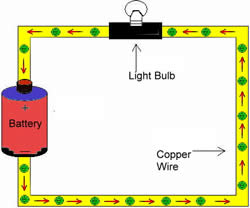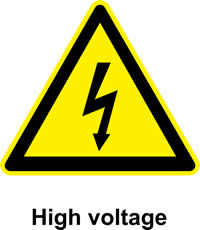# Difference between Current and Voltage

##### Key Difference: Current is the flow of electrons that pass through two voltages at different points. Voltage is the electric potential between two different points.

Current and voltage are important concepts when it comes to electricity. Though, these two are related to each other and help how electricity works, they are two different concepts. A voltage can exist without a current; however a current requires voltage to exist. Together voltage and current are part of the Ohm’s law that provides the basic backbone of electricity.Current or electric current is the flow of electrons that pass through two voltages at different points. The electrons must pass through an electric conductor to produce a charge. In a circuit, the charge is carried by electrons that are being passed through the wire or a conductor. It can also be carried by ions in an electrolyte or by both ions and electrons. Electric currents can also cause effects such as heating and magnetic fields. Ohm’s law states “that the current through a conductor between two points is directly proportional to the potential difference across the two points.” The symbol for current is ‘I’, which is derived from French phrase ‘intensité de courant’ meaning ‘current intensity’. Current is measures in ampere, named after physicist and mathematician André-Marie Ampère. Currents are measured using an ammeter.

There are two types of currents: Direct current (DC) and alternating current (AC). Direct current is when the current the electrons flow in one constant direction, such as the current produced by batteries, solar cells, etc. Alternating current is when the direction of electrons is constantly changing and is being periodically reversed. This method is used by the power companies when supplying electricity to households. Static electricity is also a form of electricity which is measured in volts.Voltage is the electric potential between two different points. It could also be used to refer to the difference in electric potential energy of a unit test charge transported between two points. A voltage may represent a source of energy or it could represent lost, used or stored energy. Voltage is the pressure of pushing electrons along in a circuit. Two paths require voltage in order for a current to pass through them. Voltage is also the total energy required to move a small electric charge between two points. Voltage is defined in such a way that negatively charged objects are pulled towards higher voltages, while positively charged voltages are pulled towards lower voltages. Volts are measured using a voltmeter.

Let’s simplify these two concepts using water as an analogy. Imagine having two tanks of water and a pipe that is placed to connect the two tanks of water. Now, the tank which has more water will automatically flow into the tank which has less water. The rate at which the water flows is similar to the flow of electrons causing a current. If the pipe that connects the two tanks was smaller, it would result in more resistance and the less water would travel; however if the pipe was wider, there would be less resistance and more water would flow from one tank to the other. This is how electricity actually works. The tanks and the air pressure pushing the water from one tank to another is the voltage, while the water which is similar to electrons is creating a current. Lastly, the pipe resembles the conductor through which the electrons travel from one voltage to another. The mathematical equation derived to represent this relationship is I = V/R, where I is the current, V is the potential difference between two point and R is the resistance, which is measured in ohms. According to Ohm’s law, the R in the relation is always constant, independent of the current.

According to experts, it is not high voltage that kills a person when they are electrocuted but the amount of current that flows through the heart. So, if the voltage is high but the current is low, there are more chances that a person would survive, while the opposite would kill the person faster. This is one of the reasons why it is believed that static electricity does not kill us. Static electricity is measured in high voltages, but it does not induce a high enough current flow.

A detailed comparison between current and voltage as on Diffen.com:

 Current Voltage Definition Current is the rate at which electric charge flows past a point in a circuit. In other words, current is the rate of flow of electric charge. Voltage, also called electromotive force, is the potential difference in charge between two points in an electrical field. In other words, voltage is the "energy per unit charge”. Symbol I V Unit A or amps or ampere V or volts or voltage SI Unit 1 ampere =1 coulomb/second. 1 volt = 1 joule/coulomb. Measuring Instrument Ammeter Voltmeter Relationship Current is the effect (voltage being the cause). Current cannot flow without Voltage. Voltage is the cause and current is its effect. Voltage can exist without current. Field created A magnetic field An electrostatic field In series connection Current is the same through all components connected in series. Voltage gets distributed over components connected in series. In a parallel connection Current gets distributed over components connected in parallel. Voltages are the same across all components connected in parallel.

Image Courtesy: etorgerson.net, timmestein.nl

Nice explanation !!!

it,s simple

simple & excellent example. nice........

simple and excellent example. nice........

now iam clear

i m confuse . when m reading electronic devices

Simply Superb

Oh very simple explanation , Thanks

omgg "yah very simple explanation nice.." hahahahahahahahaahahahahahahahahahahahahahahahahahahaahahaha you sound exactly like my chemistry teacher hahaha

Explained well with the analogy of water tank'kudos'